Sheath structure in plasma with two species of positive ions and secondary electrons
Zhao Xiao-Yun1, 2, 3, 4, Xiang Nong1, 4, †,, Ou Jing1, 4, Li De-Hui1, 4, Lin Bin-Bin1, 4
Institute of Plasma Physics Chinese Academy of Sciences (CAS) Hefei 230031, China
Science Island Branch of Graduate School, University of Science and Technology of China, Hefei 230031, China
School of Physics and Electronic Engineering, Fuyang Normal University, Fuyang 236037, China
China for Magnetic Fusion Theory, Chinese Academy of Sciences, Hefei 230031, China

† Corresponding author. E-mail: xiangn@ipp.ac.cn

Project supported by the National Natural Science Foundation of China (Grant Nos. 11475220 and 11405208), the Program of Fusion Reactor Physics and Digital Tokamak with the CAS “One-Three-Five” Strategic Planning, the National ITER Program of China (Grant No. 2015GB101003), and the Higher Education Natural Science Research Project of Anhui Province, China (Grant No. 2015KJ009).

Abstract
Abstract

The properties of a collisionless plasma sheath are investigated by using a fluid model in which two species of positive ions and secondary electrons are taken into account. It is shown that the positive ion speeds at the sheath edge increase with secondary electron emission (SEE) coefficient, and the sheath structure is affected by the interplay between the two species of positive ions and secondary electrons. The critical SEE coefficients and the sheath widths depend strongly on the positive ion charge number, mass and concentration in the cases with and without SEE. In addition, ion kinetic energy flux to the wall and the impact of positive ion species on secondary electron density at the sheath edge are also discussed.

1. Introduction

Plasma with multiple positive ion species has been found to have a wide range of applications in lab plasmas, space plasmas, and fusion plasma devices. The study of plasma sheath with multiple ion species is an important topic theoretically and experimentally since the sheath plays a very important role in determining the plasma-wall interactions and strongly affects the edge-plasma properties. For a weakly collisional sheath plasma with multiple positive ion species, Riemann pointed out that the ion velocities at the sheath edge must satisfy the Bohm criterion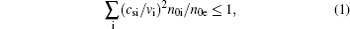where csi and vi are the ion sound speed and individual drift velocity and n0e and n0i are the electron and ion densities at the sheath edge, respectively. Apparently, an infinite number of combinations of ion velocities satisfy inequality (1). However, two extreme cases are usually considered. One is that each species satisfies its own Bohm velocity at the sheath edge. The other is that all ions reach the sheath edge with the common sound velocity. For the first case, the effects of the mass ratio and the relative concentrations of two ion species on the Bohm criterion are discussed in Refs.  and . The ion Bohm velocity increases with the increase of the charge number of positive ions.[4,7] Though a huge number of contributions have been devoted to the Bohm criterion for multiple ions, the effect of the SEE is not taken into account, since it has been found from simulations that SEE can change the Bohm criterion in a single-ion plasma; therefore, it is important to study the Bohm criterion of multiple ion species including the effect of SEE.

The characteristics of the sheath containing two single-charged ions with different mass values have been investigated by several authors.[13,1517] It is shown from these references that the two-ion-species plasma sheath is affected by ion temperature, external applied magnetic field and negative ion. However, the effect of SEE on a plasma sheath containing two ion species is still absent. For a single-ion sheath, SEE can cause the sheath potential drop and sheath width to decrease, it can even give rise to the sheath instability if the SEE coefficient reaches a critical value γc (defined as the value when the electric field equals zero at the wall). For γ > γc, the sheath structure becomes complex.

In this work, we will investigate a sheath of plasma with two species of positive ions and secondary electrons. In Section 2, the fluid equations are described. In Section 3, the Bohm criterion and the results of the analysis of the model are presented. In Section 4, conclusions are given.

2. Governing equations

In this section, we consider a collisionless plasma model containing electrons, two species of positive ions, and secondary electrons emitted from the wall. As shown in Fig. 1, the coordinate origin x = 0 is defined as the plasma-sheath boundary, x < 0 is the bulk plasma region and x > 0 is the plasma sheath region.

 Figure Option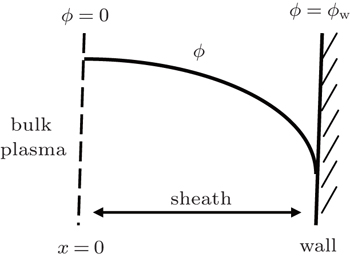Fig. 1. Schematic diagram of plasma sheath.

The electron density in the sheath can be described by the Boltzmann distribution in the thermodynamic equilibrium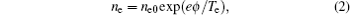where ne and Te are the density and temperature of primary electrons, respectively. ne0 is the primary electron density at the sheath edge, and e is the elementary electron charge.

In the steady state, for a collisionless plasma sheath, the continuity and momentum transport equations for cold ions are described as follows: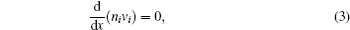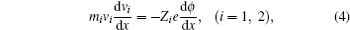where mi, ni, Zi, and vi are the mass, density, charge number and velocity of the i-th positive ion, respectively. The subscript i = 1, 2 denotes single- and multi-charged ion, respectively.

When primary electrons impinge on the solid material, secondary electrons can be emitted from the wall. Usually, the ion-inducted SEE is small compared with the electron-induced SEE, and the ion-inducted SEE is ignored unless ion impact energies of Ti ≥ 1 keV.[26,27] Secondary electrons emitted from the wall are low in energy due to the interplay between primary electrons and the wall. The peak of energy distribution of secondary electrons from the wall is generally on the order of a few electronvolts. Thus secondary electrons in the sheath can be assumed to follow the conservation of flux and energy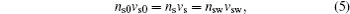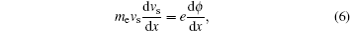where ns and vs are the density and velocity of secondary electrons, respectively. The subscripts “0”and “w” denote the locations at the sheath edge and the wall, respectively.

According to Eqs. (5) and (6), the density of secondary electrons in the sheath can be written as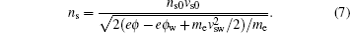For a steady plasma, the condition of zero current at the wall, determining the flux balance, is written as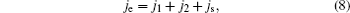where j is the flux density. ji, je, and js can be given, respectively, by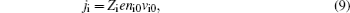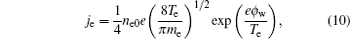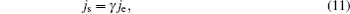where γ is the SEE coefficient.

Charge neutrality at the sheath edge x = 0 is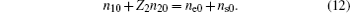The electrostatic potential is determined by the Poisson equation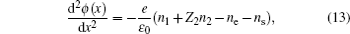where ε0 is the permittivity of free space.

For convenience, we introduce the dimensionless variables as follows: ξ = x/λDe, φ = /Te, Ne,i,s = ne,i,s/ne0, ui = vi/cs1, usw = vsw/cs1, and μ = m1/me, where λDe = [ε0Te/(ne0e2)]1/2 is the electron Debye length and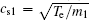is the first species ion sound speed. With these variables the model equations can be rewritten in the following dimensionless form: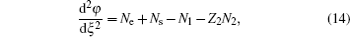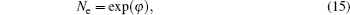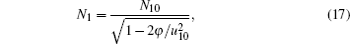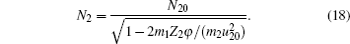In addition, by combining Eqs. (11) and (12), one can obtain the following equation: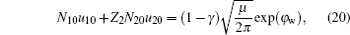where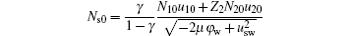is the dimensionless density of secondary electrons at the sheath edge.

3. Numerical results and discussion

In this section, the sheath characteristics are investigated numerically. For clarity, we assume that the first ion species, Ar+ is the main component of positive ion in plasma. At the sheath edge, ξ = 0, φ = 0 and the edge electric field E0 = − dφ/dξ |ξ=0 = 0.01 is set instead of zero due to the more numerical stable solutions since the sheath structure in the case E0 ≪ 1 is very similar to that in the case E0 = 0.[17,28,29] At the wall, the normalized velocity of secondary electrons is assumed to be usw = 5. The boundary velocity u10 can be determined by the sheath criterion.

3.1. Sheath criterion

The ion velocities at the sheath edge, containing two positive ion species, have been investigated by many authors. The Bohm criterion for the sheath, is still an open issue. Especially, the two-stream instability has been mentioned in recent years. The two-stream instability is not observed in the parameter range explored and each ion species satisfies its individual Bohm criterion for a collisionless plasma.[69,30] So in the absence of ion-ion streaming instability, we can assume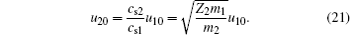The ion velocity at the sheath edge will be affected in the presence of SEE, from Eq. (14) we have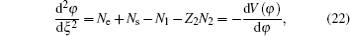where V(φ) is called the Sagdeev potential. At the sheath edge, the Sagdeev potential satisfies the boundary conditions V|φ=0 = 0 and ∂V/∂φ|φ=0 = 0. Combining Eqs. (14)–(18) with Eq. (22) and relation d2V/dφ2|φ=0 ≤ 0, we can obtain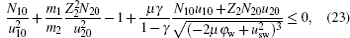When SEE is neglected, equation (23) gives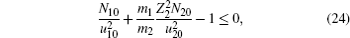which is consistent with that in Ref. . Assuming N20 = 0 in inequality (24), the Bohm criterion is u10 ≥ 1, which is the usual Bohm criterion for cold ions.

When SEE is taken into consideration in two-ion-species plasma, the critical value of ion velocity at the sheath edge u10 can be obtained from Eqs. (19)–(21), and (23) for the given parameter N20. Figure 2(a) shows that the critical value of u10 increases monotonically with SEE coefficient γ for each of Ar–He, Ar, and Ar–Xe plasmas while N20 = 0.1. Especially no matter what kind of positive ion is contained in the plasma, the effect of SEE on the boundary ion velocity is very obvious when the SEE coefficient approaches to 1. The different species of ion only slightly modifies the critical ion velocity when N20 = 0.1. Figure 2(b) represents that the critical ion velocity at the sheath edge depends on the ion mass ratio when the SEE coefficient equals γc. The critical value of ion velocity increases with the rising of the ion mass ratio. Comparing Fig. 2(a) with Fig. 2(b), we can see that the SEE coefficient influences the Ar–He plasma sheath most significantly for each of the Ar–He, Ar and Ar–Xe plasmas when γ < γc; however, the critical ion velocity in the Ar–Xe plasma is the greatest in the three cases when γ = γc in Fig. 2(b). The reason for this is that the critical SEE coefficient is related to the ion species.

 Figure Option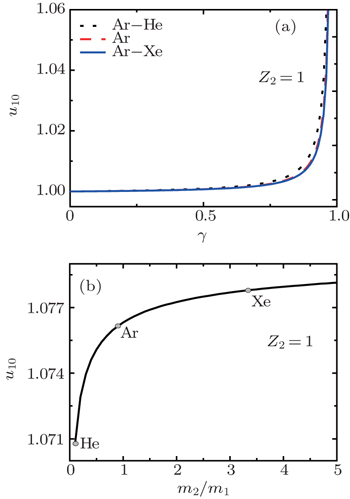Fig. 2. Variations of critical ion velocity u10 at the sheath edge with (a) SEE coefficient γ for different plasma (Ar–He, Ar, and Ar–Xe) and (b) ion mass ratio for γ = γc while N20 = 0.1.
3.2. Wall potential

The effects of ion mass ratio, SEE coefficient and the density and charge number of the second ion species on the wall potential are shown in Fig. 3. It is seen that the wall potential decreases (the sheath potential drop increases) with the increase of ion mass ratio m2/m1 and increases with the rising of the charged number of another positive ion Z2. In addition, figure 3(a) shows that the more the number density of the heavier ion (m2/m1 > 1) at the sheath edge, the lower the wall potential is. For the lighter ion (m2/m1 < 1), the opposite is the case. The result is the same as that of Ref. . Figure 3(b) represents that the wall potential rises with increasing the number density of the multi-charged ion. Meanwhile, the SEE coefficient can cause the wall potential to increase (the sheath potential drop to decrease) in plasma with multi-charged ion species and the tendency is consistent with that in the single-charged ion shown in Ref. .

 Figure Option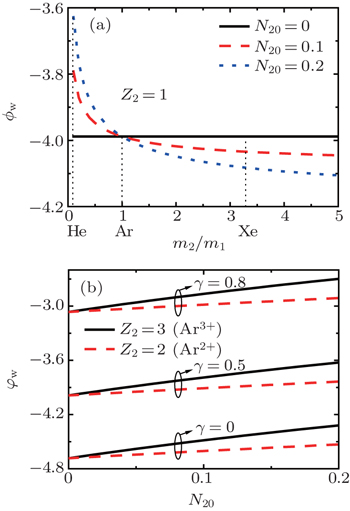Fig. 3. Variations of wall potential φw with (a) ion mass ratio m2/m1 for different values of N20, and (b) with the relative concentration of two ion species N20 for different values of Z2 and γ.
3.3. Critical SEE coefficient

The sheath potential drop will be reduced in the presence of SEE. The sheath potential drop is no longer monotonic and the sheath structure becomes complex when SEE coefficient γ is greater than γc.[19,22,23] In Ref. , the critical SEE coefficient iswhen the velocity of ion at the sheath edge is assumed to be the ion sound velocity. However, in our model, by integrating Poisson’s equation (14) over the potential using the zero electric field condition at the wall, the following equation with multiple charged positive ions can be obtained: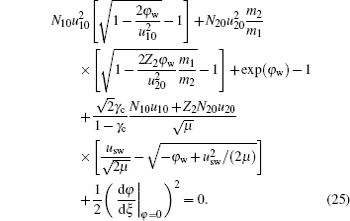The critical SEE coefficient, γc can be found from Eqs. (19)–(21), (23), and (25). Figure 4 shows the critical value of SEE coefficient with the relative concentration between two ion species. In Fig. 4(a), it is shown that the critical coefficient γc increases when the relative ion concentration for nXe+/ne0 increases in the argon plasma. The critical coefficient decreases with the rising of ion concentration for nHe+/ne0. From Fig. 4(a), one can see that for N20 = 0.2 the critical SEE coefficient reaches values of 0.973 in the Ar–Xe plasma and 0.959 in the Ar–He plasma, respectively. However, the critical coefficient is 0.97 in the pure Ar plasma. It indicates that the critical coefficient in the heavier plasma is greater than that in the lighter plasma. Figure 4(b) represents that the critical coefficient decreases when the charge number and the density of multi-charged positive ions increase. At N20 = 0.2, the critical SEE coefficient has values of 0.966 for Z2 = 2 and 0.959 for Z2 = 3, respectively. The fact that the lighter mass ion has a smaller critical SEE coefficient is because it has a smaller wall electric field without SEE. Hence it needs a smaller SEE coefficient to satisfy the condition that the wall electric field equals zero.

 Figure Option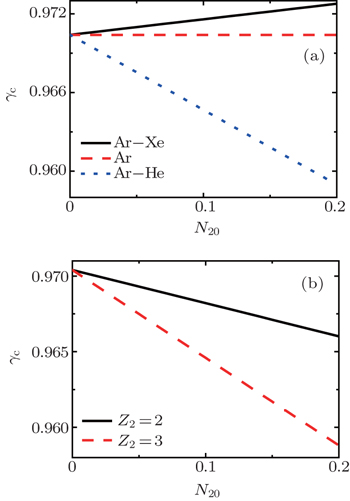Fig. 4. Variations of critical value of SEE coefficient with relative concentration between two ion species for different (a) ion species at Z2 = 1 and (b) charge number Z2 = 2 (Ar2+) and 3 (Ar3+).
3.4. Sheath width

By integrating Poisson’s equation (14) twice over the potential, the following equation can be obtained: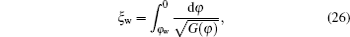where G(φ), a function of the sheath potential, is expressed as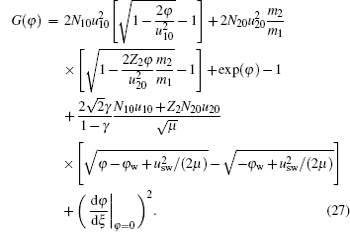The wall potential, φw can be found from Eqs. (19)–(21) and (23) for the given parameters γ and N20. Then the sheath width ξw can be obtained from Eq. (26).

Figure 5 shows the normalized sheath widths as a function of the relative concentration between two ion species with different species and the charge number of positive ion for γ = 0, γ = 0.5, and γ = 0.8. The sheath width increases with the rising of ion concentration for nXe+/ne0 in the argon plasma and decreases with the rising of ion concentration for nHe+/ne0. It means that the presence of heavier ion increases the sheath width in lighter ion plasma. The heavier ion can cause the wall potential to decrease (see Fig. 3(a)). According to Eq. (26), the increase of the sheath width is obtained. In Fig. 5(b), we can see that the rising of charge number and the density of multi-charged ions can also cause the sheath width to decrease. This is because both the heavier ion and less charge number ions are slower in velocity, the wall potential decreases and the sheath width increases. In addition, it also shows that SEE reduces the sheath width in plasmas containing multi-charge positive ions in Fig. 5, and this result is consistent with that of single-charged ion plasma in Ref. .

 Figure Option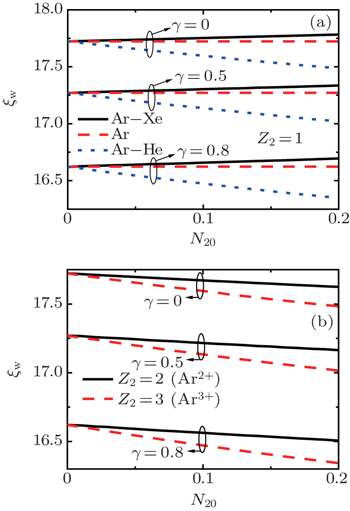Fig. 5. Variations of sheath width ξw with relative concentration between two ion species for different (a) ion species (b) charge numbers with γ = 0, γ = 0.5, and γ = 0.8.
3.5. Density of secondary electrons at the sheath edge

Secondary electrons emitted from the wall will move to the bulk plasma due to the action of the sheath electric field. The effective electron temperature in the bulk plasma will reduce when the density of secondary electrons increases. From Figs. 6(a) and 6(b) we can see that the density of secondary electrons at the sheath edge varies with the addition of another positive ion in the argon plasma. In Fig. 6(a), one can see that secondary electron density at the sheath edge increases in the presence of He+ and decreases in the presence of Xe+ as compared with the scenario in the pure argon plasma. Also it can be seen from Fig. 6(b), that the secondary electron density increases with the rising of both the charge number and the density of multi-charged ions. For example, compared with a single-charge ion species (N20 = 0), the secondary electron density has increased rates of 18% for Z2 = 2 and 50% for Z2 = 3 respectively when the relative concentration of multiple charge ions is N20 = 0.2. The reason for this phenomenon is as follows: the sheath potential increases in the presence of lighter ions (see Fig. 3). Therefore, electrons arriving at the wall are higher in energy and more secondary electrons can be emitted from the wall. Also, the bigger the charge number of ions, the faster the movement toward the wall for ions is. So the wall potential will increase, and the density of primary electrons hitting the wall and the density of secondary electrons emitted from the wall both increase.

 Figure Option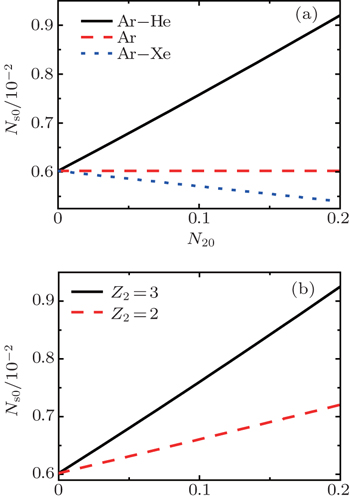Fig. 6. Secondary electron densities at the sheath edge varying with relative concentration between two ion species for different (a) ion species with Z2 = 1 (b) charge number Z2 = 2 (Ar2+) and Z2 = 3 (Ar3+) for γ = 0.8.
3.6. Ion kinetic energy flux to the wall

The ion kinetic energy flux to the wall plays an important role in plasma processing.[33,34] The ions are accelerated by the electric field of the sheath. Thus the ions arriving at the wall will have very large energy. The kinetic energy flux of ions Q can be expressed as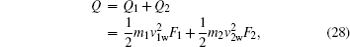where F1 and F2 are the particle fluxes of two ion species, respectively. The normalized total ion kinetic energy flux to the wall is given by ne0cs1Te, and we have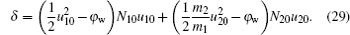The normalized ion kinetic energy fluxes are plotted in Figs. 7(a) and 7(b). The ion kinetic energy flux to the wall depends on SEE coefficient, ion species and charge number. SEE leads to a decrease in ion kinetic energy flux to the wall, even in the presence of multi-charged ions. Compared with the case of γ = 0, the ion kinetic energy flux has reduced rates of 13% for γ = 0.5 and 30% for γ = 0.8 respectively. The effect of SEE on the ion kinetic energy flux to the wall stems from the sheath potential drop. The sheath potential drop decreases with the rising of SEE coefficient (see Fig. 3). According to formula (29), the result is obtained. Meanwhile, the ion kinetic energy flux to the wall increases when there is a small amount of He+ in the argon plasma as shown in Fig. 7(a). However, the presence of Xe+ reduces the kinetic energy flux. As one sees from Fig. 7(b), with the rising of charge number, the density of multi-charged positive ions reduces the ion kinetic energy flux to the wall. According to Eq. (19), the density of singly charged positive ions will reduce when the density and the charge number of multi-charge ions increases. Combining formula (29), we can obtain the results of Fig. 7(b).

 Figure Option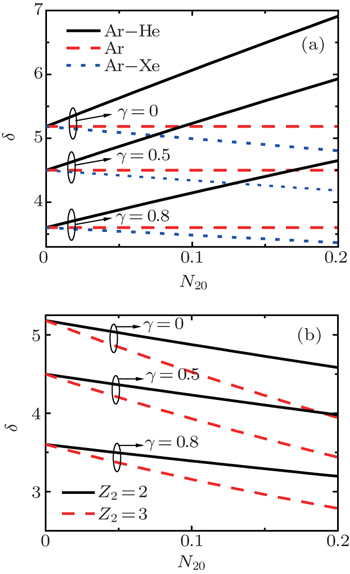Fig. 7. Normalized ion kinetic energy fluxes to the wall as a function of relative concentration between two ion species with (a) ion species for Z2 = 1 and (b) charge number Z2 = 2 (Ar2+) and Z2 = 3 (Ar3+) for γ = 0, γ = 0.5, and γ = 0.8.
4. Summary and discussion

By using a simple sheath model, we study a collisionless sheath structure of plasma consisting of electrons, two species of positive ions and secondary electrons. Based on the ion wave approach, a Bohm criterion including the effect of SEE is obtained theoretically by introducing the Sagdeev potential. It is shown that the critical ion velocity at the sheath edge increases with the SEE coefficient and the tendency is independent of ion species. Since some sheath parameters can be obtained from the plasma parameters at the sheath edge, we investigate the effects of SEE coefficient, ion species concentration and charge number on the sheath parameters such as sheath width and ion kinetic energy flux to the wall without calculating plasma parameters profiles inside the sheath. Our results show that SEE can reduce the sheath potential drop, the sheath width and ion kinetic energy flux to the wall in plasmas with multi-charged ions. Meanwhile, in the presence of lighter ion species, secondary electron density at the sheath edge and ion kinetic energy flux to the wall increase, while the critical emission coefficient and the sheath width decrease. The more the lighter positive ion concentration, the more obvious the variation is. For the presence of the heavier ion species, their variation is opposite. In addition, the increase in the charge number of ions will reduce the critical SEE coefficient, the ion kinetic energy flux to the wall and the sheath width and increase the secondary electron density at the sheath edge.

Reference
 1 Lieberman M ALichtenberg A J2005Principles of Plasma Discharges and Materials ProcessingNew YorkWiley 2 Barjatya ASwenson C MThompson D CWright K H 2009 Rev. Sci. Instrum. 80 041301 3 Xu X QUmansky M VDudson BSnyder P B2008Commun. Comput. Phys.4949 4 Riemann K U 1995 IEEE Trans. Plasma Sci. 23 709 5 Lee DHershkowitz NSevern G 2007 Appl. Phys. Lett. 91 041505 6 Gudmundsson J TLieberman M A2011Phys. Rev. Lett.107035009 7 Xiang NHu Y MOu J 2011 Plasma Sci. Technol. 13 385 8 Franklin R N 2001 Plasma Sources Sci. Technol. 10 162 9 Franklin R N 2003 J. Phys. D: Appl. Phys. 36 R309 10 Severn G DXu WEunsuk KHershkowitz N 2003 Phys. Rev. Lett. 90 145001 11 Baalrud SHegna CCallen J 2009 Phys. Rev. Lett. 103 205002 12 Yip C SHershkowitz N 2010 Phys. Rev. Lett. 104 225003 13 Khoramabadi MGhomi HShukla P K 2013 J. Plasma Phys. 79 267 14 Zhao X YLiu J YDuan PNi Z X2011Acta Phys. Sin.60045205(in Chinese) 15 Hatami M MNiknam A RShokri BGhomi H 2008 Phys. Plasmas 15 053508 16 Hatami M M 2013 Phys. Plasmas 20 013509 17 Hatami M M 2015 Phys. Plasmas 22 043510 18 Hobbs G DWesson J 1967 Plasma Phys. 9 85 19 Schwager L A 1993 Phys. Fluids B 5 631 20 Ahedo E 2002 Phys. Plasmas 9 4340 21 Gyergyek TKovačič JČerček M 2010 Contrib. Plasma Phys. 50 121 22 Sheehan J PHershkowitz NKaganovich I DWang HRaitses YBarnat E VWeatherford B RSydorenko D 2013 Phys. Rev. Lett. 111 075002 23 Zhang F KDing Y JQing S WWu X D 2011 Chin. Phys. B 20 125201 24 Yu D RQing S WYan G JDuan P 2011 Chin. Phys. B 20 065204 25 Duan PQin H JZhou X WCao A NChen LGao H 2014 Chin. Phys. B 23 075203 26 Raizer Y P1991Gas Discharge PhysicsBerlinSpringer 27 Stangeby P C2000The Plasma Boundary of Magnetic Fusion DevicesBristolIOP Publishingp. 646 28 Pandey B PSamarian AVladimirov S V 2008 Plasma Phys. Control Fusion 50 055003 29 Khoramabadi MMasoudi S F 2013 Chin. Phys. Lett. 30 085202 30 Čerček MFilipič GGyergyek TKovačič J 2010 Contrib. Plasma Phys. 50 909 31 Chen F F1974Introduction to Plasma PhysicsNew YorkPlenump. 156 32 Bohm D1949The Characteristics of Electrical Discharges in Magnetic FieldsNew YorkMcGraw-Hill 33 Buzzi F LTing Y HWendt A E 2009 Plasma Sources Sci. Technol. 18 025009 34 Campanell M D 2015 Phys. Plasmas 22 040702